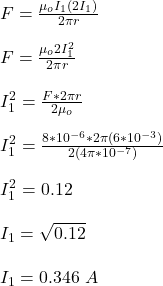## Two long parallel wires are separated by 6.0 mm. The current in one of the wires is twice the other current. If the magnitude of the force o

Question

Two long parallel wires are separated by 6.0 mm. The current in one of the wires is twice the other current. If the magnitude of the force on a 3.0-m length of one of the wires is equal to 8.0 μN, what is the greater of the two currents?

in progress 0
5 months 2021-08-05T17:36:34+00:00 1 Answers 15 views 0

## Answers ( )

The greater of the two currents is 0.692 A

Explanation:

Given;

distance between the two parallel wires; r = 6 mm = 6 x 10⁻³ m

let the current in the first wire = I₁

then, the current in the second wire = 2I₁

length of the wires, L = 3.0 m

magnitude of force on the wires, F = 8 μN = 8 x 10⁻⁶ N

The magnitude of force on the two parallel wires is given by;the current in the second wire = 2I₁ = 2 x 0.346 A = 0.692 A

Therefore, the greater of the two currents is 0.692 A